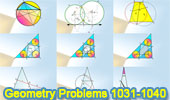# Geometry Problems 1031-1040: Triangle, Circle, Quadrilateral. Level: High School, College

 Geometry Problems 1031 - 1040Geometry Problem 1031. Trapezoid, Intersecting Circles, Common Chord, Tangent Line, Midpoint, Parallel Lines. Geometry Problem 1032: Apollonius Problem LPP. Circle Tangent to One Line and passing through Two Points. Geometry Problem 1033. Triangle, Angle Obtuse, Circumcircle, Diameter, Area. Geometry Problem 1034. Triangle, Three equal Incircles, Tangent lines, Isoperimetric triangles, Equal perimeter. Geometry Problem 1035. Triangle, Three equal Incircles, Tangent lines, Equivalent triangles, Equal area. Geometry Problem 1036. Triangle, Three equal Incircles, Tangent lines, Inradius, Equal Inradii. Geometry Problem 1037. Triangle, Three equal Incircles, Tangent lines, Inradius, Length. Geometry Problem 1038. Isosceles Triangle, Angle, 80, 20, 25, 65 Degrees. Art of Geometry Problem 1038. Using iPad Apps. Typography of Geometry Problem 1038. Using iPad Apps. Geometry Problem 1039. Isosceles Triangle, Circle, Tangent Line, Parallel Line. Geometry Problem 1040. Isosceles Triangle, Transversal Line, Metric Relations.

Home | Sitemap | Search | Geometry | Problems | Visual Index | 10 ProblemsEmail | Post a comment | by Antonio Gutierrez
Last updated Feb 16, 2018